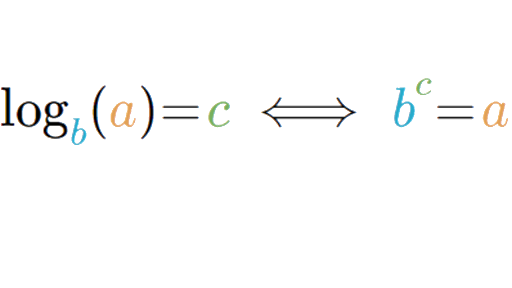What is the value of x if Y=log x & If y=10? - ProProfs DiscussTopicsMoreProductsMore+ Ask Question

# What is the value of x if Y=log x & If y=10?

This question is part of Some Hard Math Questions quiz
Asked by BRANDMAN, Last updated: Feb 20, 2020

###Request 0FollowShareAnswer AnonymouslyAnswer LaterCopy Link1

Anonymous

Sorry but I think the answer should be 10^10.1#### akonwiebrayan

Akonwiebrayan#### T. Moore

Have keen interest in writing, traveller by heart.

T. Moore, Writer, MA, Washington

The correct answer to this question is 10^10. This type of question would be primarily found on a math test. Inverse functions have one to one. Therefore, if a is the inverse of b, then b is the inverse of a. Being that they are inverses of each other, they are also one to one functions.

The domains and range are also equal to each other. Therefore, a is the domain equal to b, and then a range is equal to the range of b. As shown in the illustration provided, inverse fractions can be graphed to understand them further.AnonymousSearch for Google imagesSelect a recommended image
Upload from your computerCancelSearch for Google imagesSelect a recommended image
Upload from your computerCancelSearch for Google imagesSelect a recommended image
Upload from your computerCancel